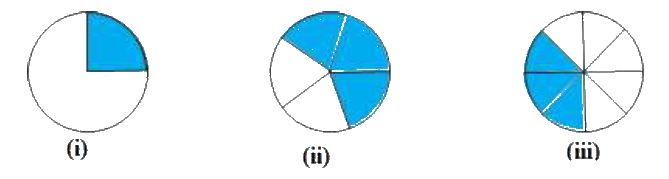# Ex.8.2 Q3 Compairing Quantities - NCERT Maths Class 7

Go back to  'Ex.8.2'

## Question

Estimate what part of the figures is coloured and hence find the per cent which is coloured.Video Solution
Comparing Quantities
Ex 8.2 | Question 3

## Text Solution

What is Known?

The part of the figures which is coloured .

What is Unknown?

What part of the figures is coloured and also the per cent which is coloured.

Reasoning:

In this question first find the the total number of parts of the figure and then the coloured parts. The fraction of coloured part is obtained by dividing number of coloured part with total umber of parts.To find the percentage multiply the given fraction by $$100.$$

Steps:

(i) In this figure, one part out of four parts is coloured. So the fraction of coloured part is \begin{align}\frac{1}{4}\end{align}. Convert the fraction into percentage by multiplying the fraction with $$100.$$

Percentage of coloured part

\begin{align}&= \frac{1}{4} \times 100 \\& = 25\% \\\end{align}

(ii) In this figure, three parts out of five parts are coloured. Hence the fraction of coloured parts is \begin{align}\frac{3}{5}\end{align}

Percentage of coloured part

\begin{align}&= \,\frac{3}{5} \times 100\\&= 60\%\end{align}

(iii) In this figure, three parts out of eight parts are coloured. Hence the fraction of coloured parts is\begin{align}\frac{3}{8}\end{align}

Percentage of coloured part

\begin{align}&= \frac{3}{8} \times 100\\&= 37.5\%\end{align}

Learn from the best math teachers and top your exams

• Live one on one classroom and doubt clearing
• Practice worksheets in and after class for conceptual clarity
• Personalized curriculum to keep up with school